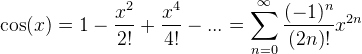# Help with matlab program for cos(x)

## Homework Statement

The function cos(x) can be approximated using the following series expansion:Write a MATLAB function called seriesCos that takes a single scalar argument, x, uses the above formula to compute cos(x), and returns the result. Your function should use a while loop and continue adding terms to the series until the absolute value of the difference between successive iterations is less than 1.e-6. MATLAB functions that may prove useful include factorial() and abs().

## Homework Equations

i can't get it to work and i'm not sure what I am doing wrong.It's not going through the loop or anything

## The Attempt at a Solution

function y=seriesCos(x);
%calculate cos(x) through a series
%user call seriesCos(x,n)
%x is number to evaluate n is desire cycles through series
x=input ('Enter x to be evalute: ');
ser=1;
sum=0;
n=1;
er=0.0;
while er>=1e-6
an=(-1)^n*x^(2*n)/(factorial(2*n));
sum=ser;
ser=sum+an;
n=n+1;
er=abs((ser-sum)/(ser));
end
disp (ser)
disp (er)

## Answers and Replies

look at the line in which you start the while loop and you should see something wrong

what is er defined to be?

CompuChip
Science Advisor
Homework Helper
You are right, it's not going through the loop, because you are telling it not to:

Code:
er=0.0;
while er>=1e-6
...

I suggest setting er to something larger than 1e-6 to start with :P